Date: Tue, 28 Oct 1997 17:26:47 +0800 Sender: Jim Subject: Multiplying imaginary numbers. Here is the question: (square root -2) x (square root -8) My algebra suggests two possibilities combine the roots under one radical getting = (square root -2 x -8) = (square root +16) = 4  or rewrite each root using i = (square root 2 i^2) x (square root 8 i^2) = i (square root 2) x i (square root 8) = i^2 (square root 16) = -1 x 4 = -4 Which answer is correct? Can you explain the reasoning? Jim Teacher, Ernie Studer School Math 30B Hi Ernie, The "square root" notation has a subtle definition that is often misunderstood. The symbol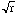stands for the one and only NON-NEGATIVE number whose square is r. The symbol makes sense only when r satisfies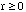. (There are other situations where restrictions are automatically built in to the notation, for example a/b means that b is nonzero because a/0 makes no sense.) Of course, every positive number r has two square roots, namelyand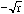. (For example, to solve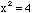one needs to find both roots, namely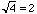and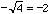.) Difficulties arise when it comes to solving the general quadratic equation. For example, the solutions to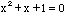are generally written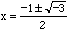. In order to make sense of a square root of a negative number we INVENT the rule that when r is positive,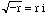, where i is one of two numbers satisfying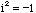. The other one is denoted by -i, but really these guys are identical twins whom nobody can tell apart. Once you allow negative numbers to have square roots, the symbolshould be avoided, particularly in computations: you lose the important rule that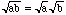. The difficulty with your problem is what is meant by (square root -2). If you mean any complex number u that satisfies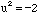then there are two choices,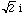and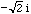. Likewise there are two solutions to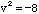,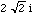and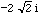. Thus (square root -2) x (square root -8) could be any of: () x () = -4 () x () = 4 () x () = 4 or () x () = -4 depending on the choices you make at any stage. Walter, Chris and Harley Go to Math Central To return to the previous page use your browser's back button.